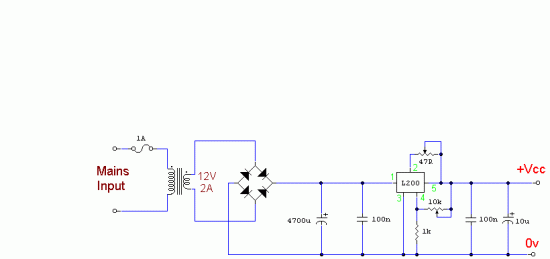## Variable Power SupplyNotes:
Using the versatile L200 voltage regulator, this power supply has independent voltage and current limits. The mains transformer has a 12volt, 2 amp rated secondary, the primary winding should equal the electricity supply in your country, which is 240V here in the UK. The 10k control is adjusts voltage output from about 3 to 15 volts, and the 47 ohm control is the current limit. This is 10mA minimum and 2 amp maximum. Reaching the current limit will reduce the output voltage to zero. Voltage and current regulation equations can be found at this page